Command Reference : Matrix Language Reference

 @colstdizep
Standardize each column using the population standard deviation.
Syntax: @colstdizep(m)
m: matrix, vector
Return: matrix, vector
Returns the matrix containing the results from standardizing each column of m.
For each element of the output: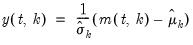for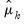the mean and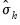the population (non d.f. corrected) standard deviation of column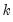where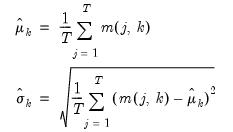(18.3)
where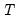is the number of non-missing values in the column. If there are missing values in a column, they are ignored and the number of rows is adjusted.
Examples
matrix m1 = @mnrnd(50, 4)
matrix m1s = @colstdizep(m1)
standardizes each column of M1 and places the results in M1D.
This operation is equivalent to
vector m1means = @cmeans(m1)
vector m1stds = @cstdevps(m1)
matrix temp = m1 - @kroncker(@ones(m1.@rows), m1means.@t)
matrix m2s = @scale(temp, m1stds.@t)
where @cmeans and @cstdevp is used to compute the column means and sample standard deviations of M1.
Cross-references